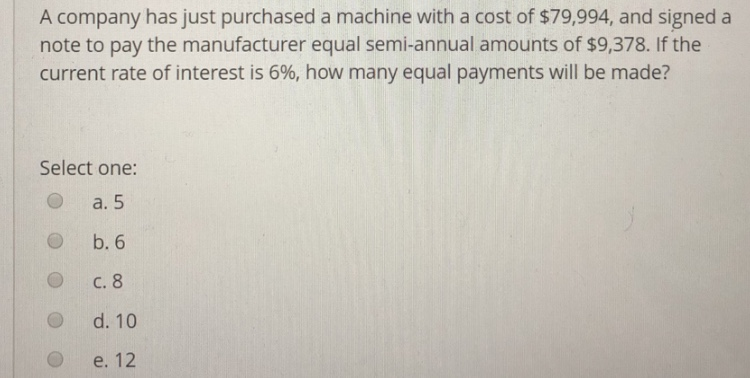# A company has just purchased a machine with a cost of \$79,994, and signed anote to pay the manufacturer equal semi-annual amounts of \$9,378. If thecurrent rate of interest is 6%, how many equal payments will be made?Select one:O a. 5O b. 6O C. 8O d. 10O e. 12

Question
504 viewshelp_outlineImage TranscriptioncloseA company has just purchased a machine with a cost of \$79,994, and signed a note to pay the manufacturer equal semi-annual amounts of \$9,378. If the current rate of interest is 6%, how many equal payments will be made? Select one: O a. 5 O b. 6 O C. 8 O d. 10 O e. 12 fullscreen
check_circle

Step 1

Present value: This is the amount of future value reduced or discounted at a rate of interest till particular current date.

Annuity: The fixed amount paid or received in equal time periods is referred to as annuity.

Step 2

Compute the number of payment periods.

Step 1: Create a new spreadsheet, save it, and input the data.

Step 2: Select cell B7 and click the function button (fx) to insert NPER function to compute number of periods.

Step 3: Click OK to select the NPER function. In the NPER function arguments window, the cursor automatically appears on the “Rate” field.

Step 4: Click on the cell B3 to select the value for “Rate” field. Since annual rate is 2 times of rate of interest, divide the value by 2.

Step 5: Place the cu...

### Want to see the full answer?

See Solution

#### Want to see this answer and more?

Solutions are written by subject experts who are available 24/7. Questions are typically answered within 1 hour.*

See Solution
*Response times may vary by subject and question.
Tagged in